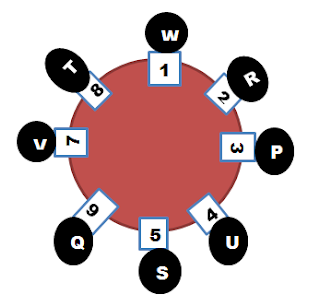# Seating Arrangement Quiz With Solution –Part 4

(Direction 1- 8): Eight Persons P, Q, R, S, T, U, V and W are sitting around a circular table facing the center but not necessarily in the same order. Each one has different weight such as 50 Kg, 54 Kg, 60 Kg, 65 Kg, 68Kg, 72 Kg, 74 Kg and 78 Kg, but not necessarily in the same order.

1. P is only greater than S
2. W, whose weight is 72Kg and One with least weight are opposite to each other
3. T is shorter than only V, who sits 3rd to the left of U
4. P and V are opposite to each other
5. Q is greater than R and Lesser than T
6. Immediate neighbour of U and Q is the one with least weight
7. R is not an immediate neighbour of V or Q
8. sum of U and T is 139
1. Which of the following is true?
A. S<P<R<U<W<Q<T<V
B. V>T>W>U>Q>R>P>S
C. V<T<Q<W<U>R>P<S
D. V>T>W>Q>U>R>P>S
E. None of these

2. What is the exact value of (P+Q)* (V+T)/(R-S)?
A. 1854.4
B. 1855.4
C. 1854.8
D. 1855.8
E. None of these

3. If all are asked to sit in alphabetic order starting from P in Anti-clockwise direction, then how many of them remain in same place?
A. None
B. One
C. Two
D. Three
E. None of these

4. Which of the following are 3rd to the right of T and 2nd to the left of U respectively?
A. P and Q
B. S and V
C. S and Q
D. P and R
E. None of these

5. Who among the following are heavier than Q?
A. T and W
B. W and U
C. V and U
D. U and T
E. None of these

6. Who is sitting between person with 60 Kg and 74 Kg?
A. Q
B. R
C. S
D. W
E. None of these

7. How many persons are sitting between P and U when counted in clockwise direction?
A. None
B. One
C. Two
D. Three
E. None of these

8. Find the odd one from the following options?
A. W and P
B. R and S
C. U and Q
D. S and V
E. V and W

Weight
Person
50 Kg
S
54 Kg
P
60 Kg
R
65 Kg
U
68 Kg
Q
72 Kg
W
74 Kg
T
78 Kg
V

#### 2. Option A

(P+Q)* (V+T)/(R-S)
(54+68)*(78+74)/(60-50)
122*152/10
18544/10
1854.4 (ans)

#### 3. Option B

P alone remains in same place

#### 4. Option CWeight
Person
50 Kg
S
54 Kg
P
60 Kg
R
65 Kg
U
68 Kg
Q
72 Kg
W
74 Kg
T
78 Kg
V

#### 6. Option D#### 7. Option A#### 8. Option B

All other have single person in between except Option B